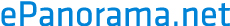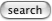# European semiconductor marking codes

European semiconductor components are quite often marked with type code which has two letters followed by 3-4 numbers (for example BC547). The first letter tells the semiconductor material and the second tells the component type group.

## First letter: semicondictor material

```A = Germanium  ( Ge )
B = Silicon  ( Si )
C = GalliumArsenide ( GaAs ) or other Gallium based
D = IndiumAntimonide ( InSb )
R = Materials for Hall-elements and photocells
```

## Second letter: component type group

```A = detector, switching or mixing diode
B = capacitance diode
C = small power low frequency transistor
D = low frequency power transistor
E = tunneling effect diode
F = small power high frequency transistor
G = combination of different components and components without own type group
H = magnetically sensitive diodes and field sensors
K = open Hall-element (detects external magnetic field)
L = high frequency power transistor
M = closed Hall-element
N = optocoupler
P = light or radiation sensing components
Q = light or radioation transmitting component
R = small power triggerable switching component
S = small power switching transistor
T = triggerable power switching component (Tristors, TRIACs etc)
U = switching power transistor
X = multiplication diode (varactor, step recovery diode)
Y = rectifying diode or similar components
Z = Zener-diode or similar components
```

# Japanise semicondicto component markings

## Japanise transistors (2Sxxx)

Japanise transistors type markignn starts typically with number 2 and letter S. That starting is followed by one letter and 2 or more numbers. The letter which follows 2S markings tells what type of transistor it is:

```A = PNP small power general purpose
B = PNP power
C = NPN small power general purpose
D = NPN power
J = P-channel fet
K = N-channel fet
```

Tomi Engdahl <[email protected]>